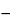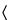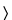Aluminum Zirconium Octachlorohydrate
(a loo' mi num zir koe' nee um ok'' ta klor'' oh hye' drate).
AlyZr(OH)3y+4xClx·nH2O
» Aluminum Zirconium Octachlorohydrate is a polymeric, loosely hydrated complex of basic aluminum zirconium chloride that encompasses a range of aluminum-to-zirconium atomic ratios between 6.0:1 and 10.0:1, and a range of (aluminum plus zirconium)-to-chloride atomic ratios between 1.5:1 and 0.9:1. It contains not less than 90.0 percent and not more than 110.0 percent of the labeled amount of anhydrous aluminum zirconium octachlorohydrate.
Packaging and storage— Preserve in well-closed containers.
Labeling— The label states the content of anhydrous aluminum zirconium octachlorohydrate.
between 3.0 and 5.0, in a solution [15 in 100 (w/w)].
Proceed as directed in the chapter, except to use the following modifications.
Test Preparation— Prepare as directed in the chapter. If the solution is not clear after dilution to 40 mL, heat for several minutes between 60and 80, and cool to room temperature. If the solution remains cloudy, repeat from the beginning with the following modification: add 3 mL of hydrochloric acid prior to adjustment of the pH with 6 N ammonium hydroxide.
Monitor Preparation— Prepare as directed in the chapter, using the same modifications described for Test Preparation if necessary.
Procedure— To each of the three tubes containing the Standard Preparation, the Test Preparation, and the Monitor Preparation, add 2 mL of pH 3.5 Acetate Buffer, and heat gently to between 60and 80. To the Standard Preparation add 6 drops of sodium sulfide TS. To the Test Preparation and the Monitor Preparation add 12 drops of sodium sulfide TS. Cool to room temperature, and dilute the contents of each tube with water to 50 mL. Gently mix each tube by inverting twice. Allow to stand for 5 minutes, and view downward over a white surface. The color of the solution from the Test Preparation is not darker than that of the solution from the Standard Preparation, and the color of the solution from the Monitor Preparation is the same as or darker than the color of the solution from the Standard Preparation. If the color of the solution from the Monitor Preparation is lighter than the color of the solution from the Standard Preparation, repeat the procedure with the following modification: after the heating step, to the Monitor Preparation and the Test Preparation add 1.0 mL, instead of 12 drops, of sodium sulfide TS. Not more than 20 µg per g is found.
Limit of iron—
Standard preparation— Transfer 2.0 mL of Standard Iron Solution, prepared as directed under Iron241, to a 50-mL beaker.
Test preparation— Transfer 2.7 g of Aluminum Zirconium Octachlorohydrate to a 100-mL volumetric flask, dilute with water to volume, and mix. Transfer 5.0 mL of this solution to a 50-mL beaker.
Procedure— To each of the beakers containing the Standard preparation and the Test preparation add 5 mL of 6 N nitric acid, cover with a watch glass, and boil on a hot plate for 3 to 5 minutes. Allow to cool, add 5 mL of Ammonium Thiocyanate Solution, prepared as directed under Iron241, transfer to separate 50-mL color comparison tubes, dilute with water to volume, and mix: the color of the solution from the Test preparation is not darker than that of the solution from the Standard preparation (150 µg per g).
Content of aluminum— Transfer about 0.15 g of Aluminum Zirconium Octachlorohydrate, accurately weighed, to a 150-mL beaker, and add 5 mL of water and 15 mL of hydrochloric acid. Heat this solution to boiling, and continue boiling for 5 minutes. Add 40 mL of water and 15.0 mL of 0.1 M edetate disodium VS. Heat the solution to boiling, and continue boiling for 5 minutes. Allow the solution to cool, add 10 to 15 mL of acetic acid–ammonium acetate buffer TS, and adjust with ammonium hydroxide to a pH of 4.5 ± 0.1. Add 20 mL of alcohol, and adjust with ammonium hydroxide to a pH of 4.6 ± 0.1. Add 5 to 10 drops of dithizone TS, and titrate with 0.1 M zinc sulfate VS until the first permanent purple-pink color appears. Perform a blank determination, and make any necessary correction. Calculate the percentage of aluminum (Al) in the Aluminum Zirconium Octachlorohydrate by the formula:
2.698[15.0 Me(zMz + Ze)] / W
in which Me is the molarity of the edetate disodium VS; z is the volume, in mL, of zinc sulfate VS consumed; Mz is the molarity of the zinc sulfate VS; W is the quantity, in g, of Aluminum Zirconium Octachlorohydrate taken; Ze is the equivalent volume, in mL, of edetate disodium VS consumed by the zirconium moiety, calculated as follows:
(Zr/Me)(W/92.97)
in which Zr is the percentage of zirconium as determined in the test for Content of zirconium, 92.97 is the atomic weight of zirconium corrected for 2% hafnium content, and the other terms are as defined above. Use the result obtained to calculate the Aluminum/zirconium atomic ratio and the (Aluminum plus zirconium)/chloride atomic ratio.
Content of zirconium— Transfer about 250 mg of Aluminum Zirconium Octachlorohydrate, accurately weighed, to a 150-mL beaker, and add 5 mL of water and 15 mL of hydrochloric acid. Heat this solution to boiling, and continue boiling for 6 to 8 minutes. Add 30 to 40 mL of water and 5 mL of hydrochloric acid, and heat to boiling. Add 1 drop of xylenol orange TS, and, while still hot, titrate with 0.1 M edetate disodium VS until the color of the solution changes from pink to yellow. Perform a blank determination, and make any necessary correction. Each mL of 0.1 M edetate disodium is equivalent to 9.297 mg of zirconium (Zr). Use the result obtained to calculate the Aluminum/zirconium atomic ratio and the (Aluminum plus zirconium)/chloride atomic ratio.
Aluminum/zirconium atomic ratio— Divide the percentage of aluminum found in the test for Content of aluminum by the percentage of zirconium found in the test for Content of zirconium, and multiply by 92.97/26.98, in which 92.97 is the atomic weight of zirconium corrected for 2% hafnium content, and 26.98 is the atomic weight of aluminum: the ratio is between 6.0:1 and 10.0:1.
Content of chloride— Transfer about 250 mg of Aluminum Zirconium Octachlorohydrate, accurately weighed, to a 250-mL beaker, add 100 to 120 mL of water and 20 mL of diluted nitric acid, and swirl to dissolve. Titrate with 0.05 N silver nitrate VS using a calomel electrode and a silver billet electrode system, determining the endpoint potentiometrically. Each mL of 0.05 N silver nitrate is equivalent to 1.773 mg of chloride (Cl). Use the result obtained to calculate the (Aluminum plus zirconium)/chloride atomic ratio.
(Aluminum plus zirconium)/chloride atomic ratio— Calculate the (aluminum plus zirconium)/chloride atomic ratio by the formula:
[(Al/26.98) + (Zr/92.97)] / (Cl/35.453)
in which Al, Zr, and Cl are the percentages of aluminum, zirconium, and chloride as determined in the tests for Content of aluminum, Content of zirconium, and Content of chloride, respectively; 26.98 is the atomic weight of aluminum; 92.97 is the atomic weight of zirconium corrected for 2% hafnium content; and 35.453 is the atomic weight of chlorine: the ratio is between 1.5:1 and 0.9:1.
Assay— Calculate the percentage of anhydrous aluminum zirconium octachlorohydrate in the Aluminum Zirconium Octachlorohydrate by the formula:
Al({26.98y + 92.97 + 17.01[3y + 4(y + 1)/z] + 35.453(y + 1) / z }/ 26.98y)
in which Al is the percentage of aluminum found in the test for Content of aluminum, y is the aluminum/zirconium atomic ratio found in the test for Aluminum/zirconium atomic ratio, z is the (aluminum plus zirconium)/chloride atomic ratio found in the test for (Aluminum plus zirconium)/chloride atomic ratio, 26.98 is the atomic weight of aluminum, 92.97 is the atomic weight of zirconium corrected for 2% hafnium content, 17.01 is the molecular weight of the hydroxide anion (OH), and 35.453 is the atomic weight of chlorine (Cl).
Auxiliary Information— Please check for your question in the FAQs before contacting USP.
Topic/Question Contact Expert Committee
Monograph Feiwen Mao, M.S.
Senior Scientific Liaison
1-301-816-8320
(SM32010) Monographs - Small Molecules 3
USP35–NF30 Page 2138
Pharmacopeial Forum: Volume No. 27(4) Page 2691